## Introduction

Estimation of an optimal holdout size (OHS) for a predictive score is a trade-off between getting a sufficiently accurate prediction and having a sufficiently large number of samples who stand to benefit from it. Intuitively, this thus requires an understanding of how much we gain (on a per-sample basis) from the predictive score being more or less accurate.

Specifically, we seek to estimate a function $$k_2(n)$$ defined as

'the expected cost per sample if using a risk score trained to $$n$$ samples'

where the expectation is over both error in cost, and in the $$n$$ training samples.

This is generally the most difficult thing to estimate in the OHS-estimation procedure. since it requires estimating the consequences of whatever action is taken in response to a given predictive score. We presume that estimation may be both expensive (in that we wish to minimise the number of values $$n$$ for which we must estimate $$k_2(n)$$) and inaccurate (in that $$\textrm{var}\{k_2(n)\}$$ may be substantial) but presume that it is unbiased.

In some cases, we may reasonably presume a parametric form for $$k_2(n)$$; namely, if the learning curve (expected precision with number of training samples) has a known form (e.g., @amari92,@amari93) as does the relation of expected cost to expected precision are known functions of $$n$$ (see example in manuscript). Other times, we may not, and learning curves may have complex behaviours [@viering21].

In this vignette we demonstrate two algorithms for estimating OHS values. One algorithm is completely parametric, using only maximum-likelihood estimates, and the other is semi-parametric, augmenting a parametric mean estimate with a Gaussian process.

Both algorithms aim to minimise the number of values $$n$$ for which $$k_2(n)$$ must be estimated. This is achieved by using a greedy algorithm to select the best 'next point' at each stage.

We will firstly demonstrate both algorithms on simulated datasets, and then demonstrate circumstances in which each is preferable to the other.

## Setup

### Assumptions throughout

We will generally parametrise the function $$k_2(n)$$ as

\begin{equation} k_2(n) = a n{-b} + c \end{equation}

governed by theta=$$\theta=(a,b,c)$$

We will consider Gaussian processes with zero mean and radial kernel

$k(n,n')=\sigma_u^2 \exp\left(-\left(\frac{n-n'}{\omega}\right)^2\right)$ parametrised by k_width=$$\omega$$ and var_u=$$\sigma_u^2$$, using the values k_width=kw0=5000 and var_u=vu0=1e7

### General setup

We briefly set up general parameters for this simulation

# Set random seed
set.seed(21423)

library(OptHoldoutSize)

# Suppose we have population size and cost-per-sample without a risk score as follows
N=100000
k1=0.4

# Suppose that true values of a,b,c are given by
theta_true=c(10000,1.2,0.2)
theta_lower=c(1,0.5,0.1) # lower bounds for estimating theta
theta_upper=c(20000,2,0.5) # upper bounds for estimating theta
theta_init=(theta_lower+theta_upper)/2 # We will start from this value when finding theta

# Kernel width and variance for Gaussian process
kw0=5000
vu0=1e7

# We will presume that these are the values of n for which cost can potentially be evaluated.
n=seq(1000,N,length=300)


## Demonstration of algorithms

### Parametric assumptions

We will consider two possible forms for the true function $$k_2(n)$$. One is a simple power-law curve (the function powerlaw() generates expected values of $$k_2(n)$$ according to the power-law parametrisation above). The other is a curve exhibiting 'double-descent' behaviour, which cannot be parametrised using a power law curve.

## True form of k2, option 1: parametric assumptions satisfied (true)
true_k2_pTRUE=function(n) powerlaw(n,theta_true)

## True form of k2, option 2: parametric assumptions NOT satisfied (false)
true_k2_pFALSE=function(n) powerlaw(n,theta_true) + (1e4)*dnorm(n,mean=4e4,sd=8e3)

## Plot
plot(0,type="n",xlim=range(n),ylim=range(true_k2_pTRUE(n)),
xlab="n",ylab=expression(paste("k","(n)")))

lines(n,true_k2_pTRUE(n),type="l",col="black")
lines(n,true_k2_pFALSE(n),type="l",col="red")
legend("topright",c("Power law", "Not power law"),col=c("black","red"),lty=1,bty="n")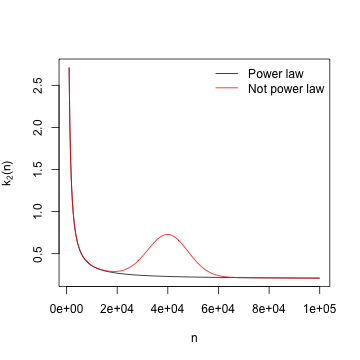We note that the double-descent form of $$k_2$$ does not satisfy the assumptions of theorem 1 in our manuscript, and hence a well-behaved single optimal holdout set size is not guaranteed. The egregious failure of parametrisation is for illustrative purposes only. A failure of parametric assumptions will lead to inconsistency in OHS estimation if the parametric approach is used, even in $$k_2$$ is only subtly misparametrised and does satisfy the assumptions of theorem 1; for instance, exponential decay parametrised using a power-law curve.

### Simulate data

We simulate a set of values $$n$$ called nset, at which unbiased estimates d are made of values $$k_2(n)$$, with independent errors with variances var_k2. We simulate two sets of values d: firstly, k2_pTRUE using the version of $$k_2(n)$$ satisfying parametric assumptions, and k2_pFALSE using the version of $$k_2(n)$$ which does not.

nsamp=200 # Presume we have this many estimates of k_2(n), between 1000 and N
vwmin=0.001; vwmax=0.02 # Sample variances var_k2 uniformly between these values

nset=round(runif(nsamp,1000,N))
var_k2=runif(nsamp,vwmin,vwmax)
k2_pTRUE=rnorm(nsamp,mean=true_k2_pTRUE(nset),sd=sqrt(var_k2))
k2_pFALSE=rnorm(nsamp,mean=true_k2_pFALSE(nset),sd=sqrt(var_k2))


The true optimal holdout sizes under the two forms of $$k_2(n)$$ are

nc=1000:N

true_ohs_pTRUE=nc[which.min(k1*nc + true_k2_pTRUE(nc)*(N-nc))]
true_ohs_pFALSE=nc[which.min(k1*nc + true_k2_pFALSE(nc)*(N-nc))]

print(true_ohs_pTRUE)
#>  27254

print(true_ohs_pFALSE)
#>  17981


We now make estimates of the optimal holdout size using the parametric and semi-parametric (Bayesian emulation) methods. Also see documentation for functions powersolve_se(), ci_ohs and error_ohs_emulation for details and examples of error estimation for such estimates.

# Estimate a,b, and c from values nset and d
est_abc_pTRUE=powersolve(nset,k2_pTRUE,
lower=theta_lower,upper=theta_upper,init=theta_init)$par est_abc_pFALSE=powersolve(nset,k2_pFALSE, lower=theta_lower,upper=theta_upper,init=theta_init)$par

# Estimate optimal holdout sizes using parametric method
param_ohs_pTRUE=optimal_holdout_size(N,k1,theta=est_abc_pTRUE)$size param_ohs_pFALSE=optimal_holdout_size(N,k1,theta=est_abc_pFALSE)$size

# Estimate optimal holdout sizes using semi-parametric (emulation) method
emul_ohs_pTRUE=optimal_holdout_size_emulation(nset,k2_pTRUE,var_k2,theta=est_abc_pTRUE,N=N,k1=k1)$size emul_ohs_pFALSE=optimal_holdout_size_emulation(nset,k2_pFALSE,var_k2,theta=est_abc_pFALSE,N=N,k1=k1)$size

# In the parametrised case, the parametric model is better
print(true_ohs_pTRUE)
#>  27254
print(param_ohs_pTRUE)
#>  27892.14
print(emul_ohs_pTRUE)
#>  36537.17

# In the misparametrised case, the semi-parametric model is better
print(true_ohs_pFALSE)
#>  17981
print(param_ohs_pFALSE)
#>  39313.83
print(emul_ohs_pFALSE)
#>  20721.51


We resample d repeatedly and recalculate the OHS with each method in order to assess the variance of these estimates.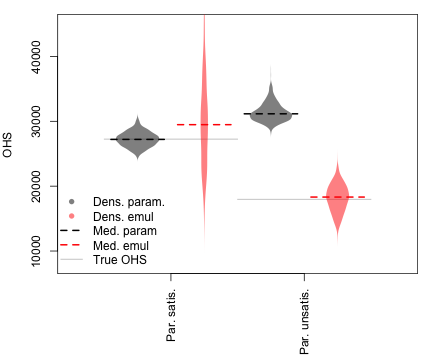Violin plots on the left show OHS estimations when parametric assumptions are satisifed, and on the right when they are unsatisfied. Note that the parametric OHS estimate is essentially unbiased and has less variance than the emulation estimate when parametric assumptions are satisfied, but is badly biased when parametric assumptions are unsatisfied. The variance of the resampled OHS estimates using the emulation method is lower when parametric assumptions are unsatisfied because the true cost function has a sharper minimum in that case (see figures in subsequent section).

Because the cost function is 'flat' around the minimum in the setting where parametric assumptions are satisfied, the consequences of the high variance of the semi-parametric (emulation) estimator are minimal, as the cost is similar across a range of values near the OHS.

Detailed code is shown below (not run)

Show detailed code

n_var=1000 # Resample d this many times to estimate OHS variance

ohs_resample=matrix(NA,n_var,4) # This will be populated with OHS estimates

for (i in 1:n_var) {
set.seed(36253 + i)

# Resample values d
k2_pTRUE_r=rnorm(nsamp,mean=true_k2_pTRUE(nset),sd=sqrt(var_k2))
k2_pFALSE_r=rnorm(nsamp,mean=true_k2_pFALSE(nset),sd=sqrt(var_k2))

# Estimate a,b, and c from values nset and d
est_abc_pTRUE_r=powersolve(nset,k2_pTRUE_r,y_var=var_k2,
lower=theta_lower,upper=theta_upper,init=theta_init)$par est_abc_pFALSE_r=powersolve(nset,k2_pFALSE_r,y_var=var_k2, lower=theta_lower,upper=theta_upper,init=theta_init)$par

# Estimate optimal holdout sizes using parametric method
param_ohs_pTRUE_r=optimal_holdout_size(N,k1,theta=est_abc_pTRUE_r)$size param_ohs_pFALSE_r=optimal_holdout_size(N,k1,theta=est_abc_pFALSE_r)$size

# Estimate optimal holdout sizes using semi-parametric (emulation) method
emul_ohs_pTRUE_r=optimal_holdout_size_emulation(nset,k2_pTRUE_r,theta=est_abc_pTRUE_r,var_k2,N,k1)$size emul_ohs_pFALSE_r=optimal_holdout_size_emulation(nset,k2_pFALSE_r,theta=est_abc_pTRUE_r,var_k2,N,k1)$size

ohs_resample[i,]=c(param_ohs_pTRUE_r, param_ohs_pFALSE_r, emul_ohs_pTRUE_r, emul_ohs_pFALSE_r)

print(i)
}

colnames(ohs_resample)=c("param_pTRUE","param_pFALSE","emul_pTRUE", "emul_pFALSE")
save(ohs_resample,file="data/ohs_resample.RData")

## To draw plot:

data(ohs_resample)

d_pt=density(ohs_resample[,"param_pTRUE"])
d_et=density(ohs_resample[,"emul_pTRUE"])
d_pf=density(ohs_resample[,"param_pFALSE"])
d_ef=density(ohs_resample[,"emul_pFALSE"])

oldpar=par(mar=c(6,4,1,1))
plot(0,type="n",xlim=c(0,5),ylim=c(8000,45000),xaxt="n",ylab="OHS",xlab="")
axis(1,at=c(1.5,3.5),label=c("Par. satis.", "Par. unsatis."),las=2)

sc=1000; hsc=0.8

lines(c(0.5,2.5),rep(true_ohs_pTRUE,2),col="gray")
lines(c(2.5,4.5),rep(true_ohs_pFALSE,2),col="gray")

polygon(1+sc*c(d_pt$y,-rev(d_pt$y)),c(d_pt$x,rev(d_pt$x)),col=rgb(0,0,0,alpha=0.5),border=NA)
polygon(2+sc*c(d_et$y,-rev(d_et$y)),c(d_et$x,rev(d_et$x)),col=rgb(1,0,0,alpha=0.5),border=NA)
polygon(3+sc*c(d_pf$y,-rev(d_pf$y)),c(d_pf$x,rev(d_pf$x)),col=rgb(0,0,0,alpha=0.5),border=NA)
polygon(4+sc*c(d_ef$y,-rev(d_ef$y)),c(d_ef$x,rev(d_ef$x)),col=rgb(1,0,0,alpha=0.5),border=NA)

lines(1+hsc*c(-0.5,0.5),rep(median(ohs_resample[,"param_pTRUE"]),2),col="black",lty=2,lwd=2)
lines(2+hsc*c(-0.5,0.5),rep(median(ohs_resample[,"emul_pTRUE"]),2),col="red",lty=2,lwd=2)
lines(3+hsc*c(-0.5,0.5),rep(median(ohs_resample[,"param_pFALSE"]),2),col="black",lty=2,lwd=2)
lines(4+hsc*c(-0.5,0.5),rep(median(ohs_resample[,"emul_pFALSE"]),2),col="red",lty=2,lwd=2)

legend("bottomleft",
c("Dens. param.","Dens. emul","Med. param","Med. emul","True OHS"),
lty=c(NA,NA,2,2,1),lwd=c(NA,NA,2,2,1),bty="n",
pch=c(16,16,NA,NA,NA),col=c(rgb(0,0,0,alpha=0.5),rgb(1,0,0,alpha=0.5),"black","red","gray"))

par(oldpar)


We may be faced with the setting where we have a set of estimated $$k_2()$$ values at a set of training sizes nset, and are able to estimate a further value at some value $$n'$$, but wish to minimise the number of new estimations we must make, and hence wish our new value $$n'$$ to have the greatest possible positive effect on our OHS estimation.

If using the parametric method, we may use the function nextpoint to do this, which seeks to find the value $$n'$$ which minimises the width of the confidence interval for the OHS should $$n'$$ and an estimate of $$k_2(n')$$ be appended to nset. Since we are concerned with accurate estimation of parameters, well-spaced values in nset are generally preferred in order to estimate $$(a,b,c)$$ well.

If using the emulation method, in which we are approximating the cost function with a Gaussian process, the global shape of the cost function (governed by the parametric part of the estimator) is less important than accurate estimation of the deviance from the parametric part (governed by the Gaussian process) near the optimal holdout size. Thus rather than nset being well-spaced out, we generally want more points close to our current estimate of the optimal holdout set size. Our strategy for sampling such points is detailed in the manuscript.

The function next_n() is used to recommend a next point in the semi-parametric setting. The following plot visualises what happens as subsequent points are added: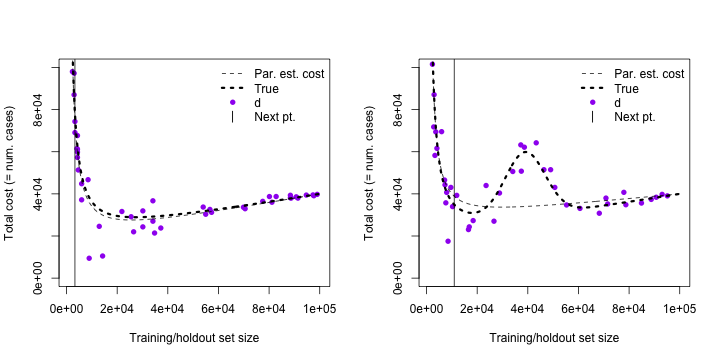Detailed code is given below

Show detailed code

## Choose an initial five training sizes at which to evaluate k2
set.seed(32424)
nstart=5
nset0=round(runif(nstart,1000,N/2))
var_k2_0=runif(nstart,vwmin,vwmax)
k2_0_pTRUE=rnorm(nstart,mean=true_k2_pTRUE(nset0),sd=sqrt(var_k2_0))
k2_0_pFALSE=rnorm(nstart,mean=true_k2_pFALSE(nset0),sd=sqrt(var_k2_0))

# These are our sets of training sizes and k2 estimates, which will be built up.
nset_pTRUE=nset0
k2_pTRUE=k2_0_pTRUE
var_k2_pTRUE=var_k2_0

nset_pFALSE=nset0
k2_pFALSE=k2_0_pFALSE
var_k2_pFALSE=var_k2_0

# Go up to this many points
max_points=200

while(length(nset_pTRUE)<= max_points) {
set.seed(37261 + length(nset_pTRUE))

# Estimate parameters
theta_pTRUE=powersolve(nset_pTRUE,k2_pTRUE,y_var=var_k2_pTRUE,lower=theta_lower,upper=theta_upper,init=theta_init)$par theta_pFALSE=powersolve(nset_pFALSE,k2_pFALSE,y_var=var_k2_pFALSE,lower=theta_lower,upper=theta_upper,init=theta_init)$par

# Find next suggested point, parametric assumptions satisfied
ci_pTRUE = next_n(n,nset_pTRUE,k2=k2_pTRUE,var_k2=var_k2_pTRUE,N=N,k1=k1,nmed=15)
if (!all(is.na(ci_pTRUE))) nextn_pTRUE=n[which.min(ci_pTRUE)] else
nextn_pTRUE=round(runif(1,1000,N))

# Find next suggested point, parametric assumptions not satisfied
ci_pFALSE = next_n(n,nset_pFALSE,k2=k2_pFALSE,var_k2=var_k2_pFALSE,N=N,k1=k1,nmed=15)
if (!all(is.na(ci_pFALSE))) nextn_pFALSE=n[which.min(ci_pFALSE)] else
nextn_pFALSE=round(runif(1,1000,N))

# New estimates of k2
var_k2_new_pTRUE=runif(1,vwmin,vwmax)
k2_new_pTRUE=rnorm(1,mean=true_k2_pTRUE(nextn_pTRUE),sd=sqrt(var_k2_new_pTRUE))

var_k2_new_pFALSE=runif(1,vwmin,vwmax)
k2_new_pFALSE=rnorm(1,mean=true_k2_pFALSE(nextn_pFALSE),sd=sqrt(var_k2_new_pFALSE))

# Update data
nset_pTRUE=c(nset_pTRUE,nextn_pTRUE)
k2_pTRUE=c(k2_pTRUE,k2_new_pTRUE)
var_k2_pTRUE=c(var_k2_pTRUE,var_k2_new_pTRUE)

nset_pFALSE=c(nset_pFALSE,nextn_pFALSE)
k2_pFALSE=c(k2_pFALSE,k2_new_pFALSE)
var_k2_pFALSE=c(var_k2_pFALSE,var_k2_new_pFALSE)

print(length(nset_pFALSE))

data_nextpoint_par=list(
nset_pTRUE=nset_pTRUE,nset_pFALSE=nset_pFALSE,
k2_pTRUE=k2_pTRUE,k2_pFALSE=k2_pFALSE,
var_k2_pTRUE=var_k2_pTRUE,var_k2_pFALSE=var_k2_pFALSE)

save(data_nextpoint_par,file="data/data_nextpoint_par.RData")

#    Sys.sleep(10)

}

data_nextpoint_par=list(
nset_pTRUE=nset_pTRUE,nset_pFALSE=nset_pFALSE,
k2_pTRUE=k2_pTRUE,k2_pFALSE=k2_pFALSE,
var_k2_pTRUE=var_k2_pTRUE,var_k2_pFALSE=var_k2_pFALSE)

save(data_nextpoint_par,file="data/data_nextpoint_par.RData")

## To draw plot with np points (np can be set using the button)

np=50 # or set using interactive session

oldpar=par(mfrow=c(1,2))
yrange=c(0,100000)

# Estimate parameters for parametric part of semi-parametric method
theta_pTRUE=powersolve(nset_pTRUE[1:np],k2_pTRUE[1:np],y_var=var_k2_pTRUE[1:np],lower=theta_lower,upper=theta_upper,init=theta_init)$par theta_pFALSE=powersolve(nset_pFALSE[1:np],k2_pFALSE[1:np],y_var=var_k2_pFALSE[1:np],lower=theta_lower,upper=theta_upper,init=theta_init)$par

## First panel
plot(0,xlim=range(n),ylim=yrange,type="n",
xlab="Training/holdout set size",
ylab="Total cost (= num. cases)")
points(nset_pTRUE[1:np],k1*nset_pTRUE[1:np] + k2_pTRUE[1:np]*(N-nset_pTRUE[1:np]),pch=16,cex=1,col="purple")
lines(n,k1*n + powerlaw(n,theta_pTRUE)*(N-n),lty=2)
lines(n,k1*n + true_k2_pTRUE(n)*(N-n),lty=3,lwd=3)
legend("topright",
c("Par. est. cost",
"True",
"d",
"Next pt."),
lty=c(2,3,NA,NA),lwd=c(1,3,NA,NA),pch=c(NA,NA,16,124),pt.cex=c(NA,NA,1,1),
col=c("black","black","purple","black"),bg="white",bty="n")

abline(v=nset_pTRUE[np+1])

## Second panel
plot(0,xlim=range(n),ylim=yrange,type="n",
xlab="Training/holdout set size",
ylab="Total cost (= num. cases)")
points(nset_pFALSE[1:np],k1*nset_pFALSE[1:np] + k2_pFALSE[1:np]*(N-nset_pFALSE[1:np]),pch=16,cex=1,col="purple")
lines(n,k1*n + powerlaw(n,theta_pFALSE)*(N-n),lty=2)
lines(n,k1*n + true_k2_pFALSE(n)*(N-n),lty=3,lwd=3)
legend("topright",
c("Par. est. cost",
"True",
"d",
"Next pt."),
lty=c(2,3,NA,NA),lwd=c(1,3,NA,NA),pch=c(NA,NA,16,124),pt.cex=c(NA,NA,1,1),
col=c("black","black","purple","black"),bg="white",bty="n")

abline(v=nset_pFALSE[np+1])

par(oldpar)


The function exp_imp_fn() is used to recommend a next point in the semi-parametric setting. The following plot visualises what happens as subsequent points are added: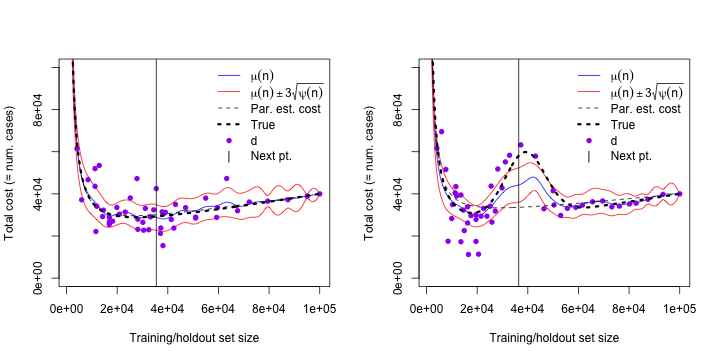Detailed code is given below

Show detailed code

## Choose an initial five training sizes at which to evaluate k2
set.seed(32424) # start from same seed as before
nstart=5
nset0=round(runif(nstart,1000,N/2))
var_k2_0=runif(nstart,vwmin,vwmax)
k2_0_pTRUE=rnorm(nstart,mean=true_k2_pTRUE(nset0),sd=sqrt(var_k2_0))
k2_0_pFALSE=rnorm(nstart,mean=true_k2_pFALSE(nset0),sd=sqrt(var_k2_0))

# These are our sets of training sizes and k2 estimates, which will be built up.
nset_pTRUE=nset0
k2_pTRUE=k2_0_pTRUE
var_k2_pTRUE=var_k2_0

nset_pFALSE=nset0
k2_pFALSE=k2_0_pFALSE
var_k2_pFALSE=var_k2_0

# Go up to this many points
max_points=200

while(length(nset_pTRUE)<= max_points) {
set.seed(46352 + length(nset_pTRUE))

# Estimate parameters for parametric part of semi-parametric method
theta_pTRUE=powersolve(nset_pTRUE,k2_pTRUE,y_var=var_k2_pTRUE,
lower=theta_lower,upper=theta_upper,init=theta_init)$par theta_pFALSE=powersolve(nset_pTRUE,k2_pFALSE,y_var=var_k2_pTRUE, lower=theta_lower,upper=theta_upper,init=theta_init)$par

# Mean and variance of emulator for cost function, parametric assumptions satisfied
p_mu_pTRUE=mu_fn(n,nset=nset_pTRUE,k2=k2_pTRUE,var_k2 = var_k2_pTRUE,theta=theta_pTRUE,N=N,k1=k1)
p_var_pTRUE=psi_fn(n,nset=nset_pTRUE,N=N,var_k2 = var_k2_pTRUE)

# Mean and variance of emulator for cost function, parametric assumptions not satisfied
p_mu_pFALSE=mu_fn(n,nset=nset_pFALSE,k2=k2_pFALSE,var_k2 = var_k2_pFALSE,theta=theta_pFALSE,N=N,k1=k1)
p_var_pFALSE=psi_fn(n,nset=nset_pFALSE,N=N,var_k2 = var_k2_pFALSE)

# Add vertical line at next suggested point
exp_imp_em_pTRUE = exp_imp_fn(n,nset=nset_pTRUE,k2=k2_pTRUE,var_k2 = var_k2_pTRUE, N=N,k1=k1)
nextn_pTRUE = n[which.max(exp_imp_em_pTRUE)]

# Find next suggested point, parametric assumptions not satisfied
exp_imp_em_pFALSE = exp_imp_fn(n,nset=nset_pFALSE,k2=k2_pFALSE,var_k2 = var_k2_pFALSE, N=N,k1=k1)
nextn_pFALSE = n[which.max(exp_imp_em_pFALSE)]

# New estimates of k2
var_k2_new_pTRUE=runif(1,vwmin,vwmax)
k2_new_pTRUE=rnorm(1,mean=true_k2_pTRUE(nextn_pTRUE),sd=sqrt(var_k2_new_pTRUE))

var_k2_new_pFALSE=runif(1,vwmin,vwmax)
k2_new_pFALSE=rnorm(1,mean=true_k2_pFALSE(nextn_pFALSE),sd=sqrt(var_k2_new_pFALSE))

# Update data
nset_pTRUE=c(nset_pTRUE,nextn_pTRUE)
k2_pTRUE=c(k2_pTRUE,k2_new_pTRUE)
var_k2_pTRUE=c(var_k2_pTRUE,var_k2_new_pTRUE)

nset_pFALSE=c(nset_pFALSE,nextn_pFALSE)
k2_pFALSE=c(k2_pFALSE,k2_new_pFALSE)
var_k2_pFALSE=c(var_k2_pFALSE,var_k2_new_pFALSE)

print(length(nset_pFALSE))

}

data_nextpoint_em=list(
nset_pTRUE=nset_pTRUE,nset_pFALSE=nset_pFALSE,
k2_pTRUE=k2_pTRUE,k2_pFALSE=k2_pFALSE,
var_k2_pTRUE=var_k2_pTRUE,var_k2_pFALSE=var_k2_pFALSE)

save(data_nextpoint_em,file="data/data_nextpoint_em.RData")

## To draw plot with np points (np can be set using the button)

np=50 # or set using interactive session

oldpar=par(mfrow=c(1,2))
yrange=c(0,100000)

# Mean and variance of emulator for cost function, parametric assumptions satisfied
p_mu_pTRUE=mu_fn(n,nset=nset_pTRUE[1:np],k2=k2_pTRUE[1:np],var_k2 = var_k2_pTRUE[1:np],N=N,k1=k1)
p_var_pTRUE=psi_fn(n,nset=nset_pTRUE[1:np],N=N,var_k2 = var_k2_pTRUE[1:np])

# Mean and variance of emulator for cost function, parametric assumptions not satisfied
p_mu_pFALSE=mu_fn(n,nset=nset_pFALSE[1:np],k2=k2_pFALSE[1:np],var_k2 = var_k2_pFALSE[1:np],N=N,k1=k1)
p_var_pFALSE=psi_fn(n,nset=nset_pFALSE[1:np],N=N,var_k2 = var_k2_pFALSE[1:np])

# Estimate parameters for parametric part of semi-parametric method
theta_pTRUE=powersolve(nset_pTRUE[1:np],k2_pTRUE[1:np],y_var=var_k2_pTRUE[1:np],lower=theta_lower,upper=theta_upper,init=theta_init)$par theta_pFALSE=powersolve(nset_pFALSE[1:np],k2_pFALSE[1:np],y_var=var_k2_pFALSE[1:np],lower=theta_lower,upper=theta_upper,init=theta_init)$par

## First panel
plot(0,xlim=range(n),ylim=yrange,type="n",
xlab="Training/holdout set size",
ylab="Total cost (= num. cases)")
lines(n,p_mu_pTRUE,col="blue")
lines(n,p_mu_pTRUE - 3*sqrt(pmax(0,p_var_pTRUE)),col="red")
lines(n,p_mu_pTRUE + 3*sqrt(pmax(0,p_var_pTRUE)),col="red")
points(nset_pTRUE[1:np],k1*nset_pTRUE[1:np] + k2_pTRUE[1:np]*(N-nset_pTRUE[1:np]),pch=16,cex=1,col="purple")
lines(n,k1*n + powerlaw(n,theta_pTRUE)*(N-n),lty=2)
lines(n,k1*n + true_k2_pTRUE(n)*(N-n),lty=3,lwd=3)
legend("topright",
c(expression(mu(n)),
expression(mu(n) %+-% 3*sqrt(psi(n))),
"Par. est. cost",
"True",
"d",
"Next pt."),
lty=c(1,1,2,3,NA,NA),lwd=c(1,1,1,3,NA,NA),pch=c(NA,NA,NA,NA,16,124),pt.cex=c(NA,NA,NA,NA,1,1),
col=c("blue","red","black","black","purple","black"),bg="white",bty="n")

abline(v=nset_pTRUE[np+1])

## Second panel
plot(0,xlim=range(n),ylim=yrange,type="n",
xlab="Training/holdout set size",
ylab="Total cost (= num. cases)")
lines(n,p_mu_pFALSE,col="blue")
lines(n,p_mu_pFALSE - 3*sqrt(pmax(0,p_var_pFALSE)),col="red")
lines(n,p_mu_pFALSE + 3*sqrt(pmax(0,p_var_pFALSE)),col="red")
points(nset_pFALSE[1:np],k1*nset_pFALSE[1:np] + k2_pFALSE[1:np]*(N-nset_pFALSE[1:np]),pch=16,cex=1,col="purple")
lines(n,k1*n + powerlaw(n,theta_pFALSE)*(N-n),lty=2)
lines(n,k1*n + true_k2_pFALSE(n)*(N-n),lty=3,lwd=3)
legend("topright",
c(expression(mu(n)),
expression(mu(n) %+-% 3*sqrt(psi(n))),
"Par. est. cost",
"True",
"d",
"Next pt."),
lty=c(1,1,2,3,NA,NA),lwd=c(1,1,1,3,NA,NA),pch=c(NA,NA,NA,NA,16,124),pt.cex=c(NA,NA,NA,NA,1,1),
col=c("blue","red","black","black","purple","black"),bg="white",bty="n")

abline(v=nset_pFALSE[np+1])

par(oldpar)


## Rates of convergence

Finally, we compare the rates of convergence of OHS estimates. The following plots show convergence rates with parametric and emulation algorithms, using either a random (black) or greedy (red) method to select the next value of $$n$$ to add to $$\mathbf{n}$$, with parametric assumptions satisfied (satis.) or unsatisfied (not satis.). Simulations are run for 200 datasets simulated independently from the underlying model. In larger panels, horizontal lines show true optimal holdout set (OHS) size; vertical lines indicate when $$\geq 2.5\%$$ of runs return that optimal holdout size within a grid of size 1000. Smaller panels show root mean-square error between total costs from simulations and minimal total cost. Note variable axis scaling on left and right.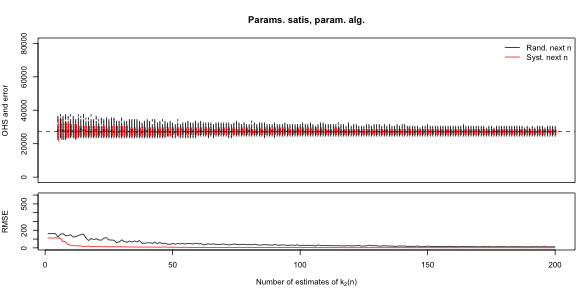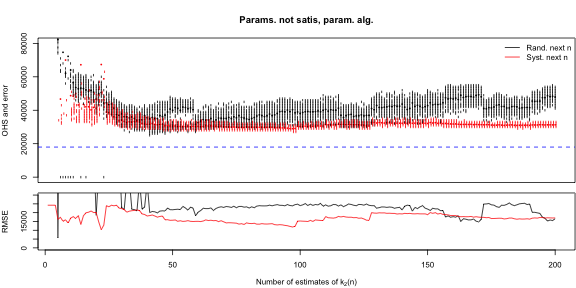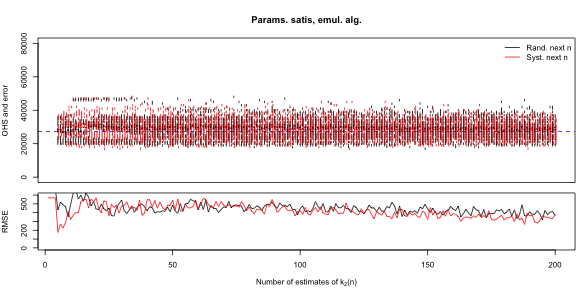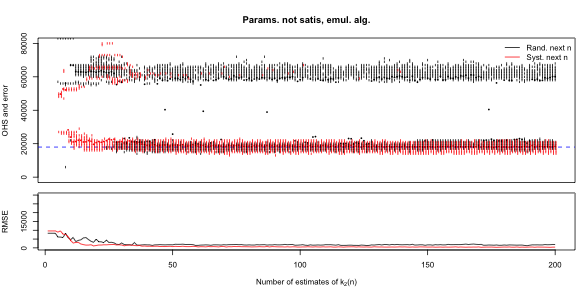We note that, in general, convergence is faster when 'next points' are picked systematically rather than randomly and convergence is faster when using parametric estimates, though as noted above parametric estimates are biased and inconsistent when parametric assumptions are not satisfied.

Detailed code is shown below (not run).

Show detailed code

# Function to resample values of d and regenerate OHS given nset and var_k2
ntri=function(nset,var_k2,k2,nx=100,method="MLE") {
out=rep(0,nx)
for (i in 1:nx) {
d1=rnorm(length(nset),mean=k2(nset),sd=sqrt(var_k2))
theta1=powersolve(nset,d1,y_var=var_k2,lower=theta_lower,upper=theta_upper,init=theta_true)$par if (method=="MLE") { out[i]=optimal_holdout_size(N,k1,theta1)$size
} else {
nn=seq(1000,N,length=1000)
p_mu=mu_fn(nn,nset=nset,k2=d1,var_k2 = var_k2, N=N,k1=k1,theta=theta1)
out[i]=nn[which.min(p_mu)]
}
}
return(out)
}

# Load datasets of 'next point'

# Maximum number of training set sizes we will consider
n_iter=200

# Generate random 'next points'
set.seed(36279)
data_nextpoint_rand=list(
nset_pTRUE=round(runif(n_iter,1000,N)),
nset_pFALSE=round(runif(n_iter,1000,N)),
var_k2_pTRUE=runif(n_iter,vwmin,vwmax),
var_k2_pFALSE=runif(n_iter,vwmin,vwmax)
)

# Initialise matrices of records
# ohs_array[n,i,j,k,l] is
#  using the first n training set sizes
#  the ith resample
#  using the jth version of k2 (j=1: pTRUE, j=2: pFALSE)
#  using the kth algorithm (k=1: parametric, k=2: semiparametric/emulation)
#  using the lth method of selecting next points (l=1: random, l=2: systematic)
nr=200 # recalculate OHS this many times
ohs_array=array(NA,dim=c(n_iter,nr,2,2,2))

for (i in 5:n_iter) {
set.seed(363726 + i)
# Resamplings for parametric algorithm, random next point
ohs_array[i,,1,1,1]=ntri(
nset=data_nextpoint_rand$nset_pTRUE[1:i], var_k2=data_nextpoint_rand$var_k2_pTRUE[1:i],
k2=true_k2_pTRUE,nx=nr,method="MLE")
ohs_array[i,,2,1,1]=ntri(
nset=data_nextpoint_rand$nset_pFALSE[1:i], var_k2=data_nextpoint_rand$var_k2_pFALSE[1:i],
k2=true_k2_pFALSE,nx=nr,method="MLE")

# Resamplings for semiparametric/emulation algorithm, random next point
ohs_array[i,,1,2,1]=ntri(
nset=data_nextpoint_rand$nset_pTRUE[1:i], var_k2=data_nextpoint_rand$var_k2_pTRUE[1:i],
k2=true_k2_pTRUE,nx=nr,method="EM")
ohs_array[i,,2,2,1]=ntri(
nset=data_nextpoint_rand$nset_pFALSE[1:i], var_k2=data_nextpoint_rand$var_k2_pFALSE[1:i],
k2=true_k2_pFALSE,nx=nr,method="EM")

# Resamplings for parametric algorithm, nonrandom (systematic) next point
ohs_array[i,,1,1,2]=ntri(
nset=data_nextpoint_par$nset_pTRUE[1:i], var_k2=data_nextpoint_par$var_k2_pTRUE[1:i],
k2=true_k2_pTRUE,nx=nr,method="MLE")
ohs_array[i,,2,1,2]=ntri(
nset=data_nextpoint_par$nset_pFALSE[1:i], var_k2=data_nextpoint_par$var_k2_pFALSE[1:i],
k2=true_k2_pFALSE,nx=nr,method="MLE")

# Resamplings for semiparametric/emulation algorithm, nonrandom (systematic) next point
ohs_array[i,,1,2,2]=ntri(
nset=data_nextpoint_em$nset_pTRUE[1:i], var_k2=data_nextpoint_em$var_k2_pTRUE[1:i],
k2=true_k2_pTRUE,nx=nr,method="EM")
ohs_array[i,,2,2,2]=ntri(
nset=data_nextpoint_em$nset_pFALSE[1:i], var_k2=data_nextpoint_em$var_k2_pFALSE[1:i],
k2=true_k2_pFALSE,nx=nr,method="EM")

print(i)

save(ohs_array,file="data/ohs_array.RData")
}

data(ohs_array)
# ohs_array[n,i,j,k,l] is
#  using the first n training set sizes
#  the ith resample
#  using the jth version of k2 (j=1: pTRUE, j=2: pFALSE)
#  using the kth algorithm (k=1: parametric, k=2: semiparametric/emulation)
#  using the lth method of selecting next points (l=1: random, l=2: systematic)

# Settings
alpha=0.5; # Plot 1-alpha confidence intervals
dd=3 # horizontal line spacing
n_iter=dim(ohs_array) # X axis range
ymax=80000 # Y axis range

# Plot drawing function
plot_ci_convergence=function(title,key,M1,M2,ohs_true,true_l) {

# Set up plot parameters
oldpar=par(mar=c(1,4,4,0.1))
layout(mat=rbind(matrix(1,4,4),matrix(2,2,4)))

# Number of estimates
n_iterx=dim(M1)

# Initialise
plot(0,xlim=c(5,n_iterx),ylim=c(0,ymax),type="n",
ylab="OHS and error",xaxt="n",main=title)
abline(h=ohs_true,col="blue",lty=2)

# Plot medians
points(1:n_iterx,rowMedians(M1,na.rm=T),pch=16,cex=0.5,col="black")
points(1:n_iterx,rowMedians(M2,na.rm=T),pch=16,cex=0.5,col="red")

# Ranges
rg1=rbind(apply(M1,1,function(x) pmax(0,quantile(x,alpha/2,na.rm=T))),
apply(M1,1,function(x) pmin(ymax,quantile(x,1-alpha/2,na.rm=T))))
rg2=rbind(apply(M2,1,function(x) pmax(0,quantile(x,alpha/2,na.rm=T))),
apply(M2,1,function(x) pmin(ymax,quantile(x,1-alpha/2,na.rm=T))))

# Coarsening factor: coarsen OHS estimates to nearest value
cfactor=1000
mfactor=5

# Record lengths of rounded OHS numbers
nrgc1=rep(dim(M1),dim(M1))
nrgc2=rep(dim(M2),dim(M2))

# Plot ranges
for (i in 1:dim(M1)) {
rgc1=cfactor*round(M1[i,]/cfactor)
t1=table(rgc1); urgc1=as.numeric(names(t1)[which(t1>mfactor)])
if (length(urgc1)<1) urgc1=NA
segments(i,urgc1-cfactor/2,
i,urgc1+cfactor/2)
if (!is.na(length(urgc1))) nrgc1[i]=length(urgc1)
}

# Plot ranges
for (i in 1:dim(M2)) {
rgc2=cfactor*round(M2[i,]/cfactor)
t2=table(rgc2); urgc2=as.numeric(names(t2)[which(t2>mfactor)])
if (length(urgc2)<1) urgc2=NA
segments(i+1/dd,urgc2-cfactor/2,
i+1/dd,urgc2+cfactor/2,
col="red")
if (!is.na(length(urgc2))) nrgc2[i]=length(urgc2)
}

legend("topright",
legend=key,bty="n",
col=c("black","red"),lty=1)

# Bottom panel setup
# Root mean square errors
rmse1=sqrt(rowMeans(true_l(M1)-true_l(ohs_true),na.rm=T)^2)
rmse2=sqrt(rowMeans(true_l(M2)-true_l(ohs_true),na.rm=T)^2)
rmse1[which(is.na(rmse1))]=max(rmse1[which(is.finite(rmse1))])
rmse2[which(is.na(rmse2))]=max(rmse2[which(is.finite(rmse2))])
rmse1=pmin(rmse1,max(max(rmse1[which(is.finite(rmse1))])))
rmse2=pmin(rmse2,max(max(rmse2[which(is.finite(rmse2))])))

par(mar=c(4,4,0.1,0.1))
plot(0,xlim=c(5,n_iterx),
ylim=c(0,ymax_lower),
type="n",ylab="RMSE",xlab=expression(paste("Number of estimates of k","(n)")))

# Draw lines
lines(1:n_iterx,rmse1,col="black")
lines(1:n_iterx,rmse2,col="red")

par(oldpar)
}

# Extract matrices from aray
M111=ohs_array[1:n_iter,,1,1,1] # pTRUE, param algorithm, random nextpoint
M112=ohs_array[1:n_iter,,1,1,2] # pTRUE, param algorithm, systematic nextpoint

M211=ohs_array[1:n_iter,,2,1,1] # pFALSE, param algorithm, random nextpoint
M212=ohs_array[1:n_iter,,2,1,2] # pFALSE, param algorithm, systematic nextpoint

M121=ohs_array[1:n_iter,,1,2,1] # pTRUE, emul algorithm, random nextpoint
M122=ohs_array[1:n_iter,,1,2,2] # pTRUE, emul algorithm, systematic nextpoint

M221=ohs_array[1:n_iter,,2,2,1] # pFALSE, emul algorithm, random nextpoint
M222=ohs_array[1:n_iter,,2,2,2] # pFALSE, emul algorithm, systematic nextpoint

# True OHS
nc=1000:N
true_ohs_pTRUE=nc[which.min(k1*nc + true_k2_pTRUE(nc)*(N-nc))]
true_ohs_pFALSE=nc[which.min(k1*nc + true_k2_pFALSE(nc)*(N-nc))]

# True functions l
l_pTRUE=function(n) k1*n + true_k2_pTRUE(n)*(N-n)
l_pFALSE=function(n) k1*n + true_k2_pFALSE(n)*(N-n)

oldpar0=par(mfrow=c(2,2))
ymax_lower=600 # Y axis range for lower plot; will vary
plot_ci_convergence("Params. satis, param. alg.",
c("Rand. next n","Syst. next n"),M111,M112,true_ohs_pTRUE,l_pTRUE)

ymax_lower=30000 # Y axis range for lower plot
plot_ci_convergence("Params. not satis, param. alg.",
c("Rand. next n","Syst. next n"),M211,M212,true_ohs_pFALSE,l_pFALSE)

ymax_lower=600 # Y axis range for lower plot; will vary
plot_ci_convergence("Params. satis, emul. alg.",
c("Rand. next n","Syst. next n"),M121,M122,true_ohs_pTRUE,l_pTRUE)

ymax_lower=30000 # Y axis range for lower plot
plot_ci_convergence("Params. not satis, emul. alg.",
c("Rand. next n","Syst. next n"),M221,M222,true_ohs_pFALSE,l_pFALSE)

par(oldpar)